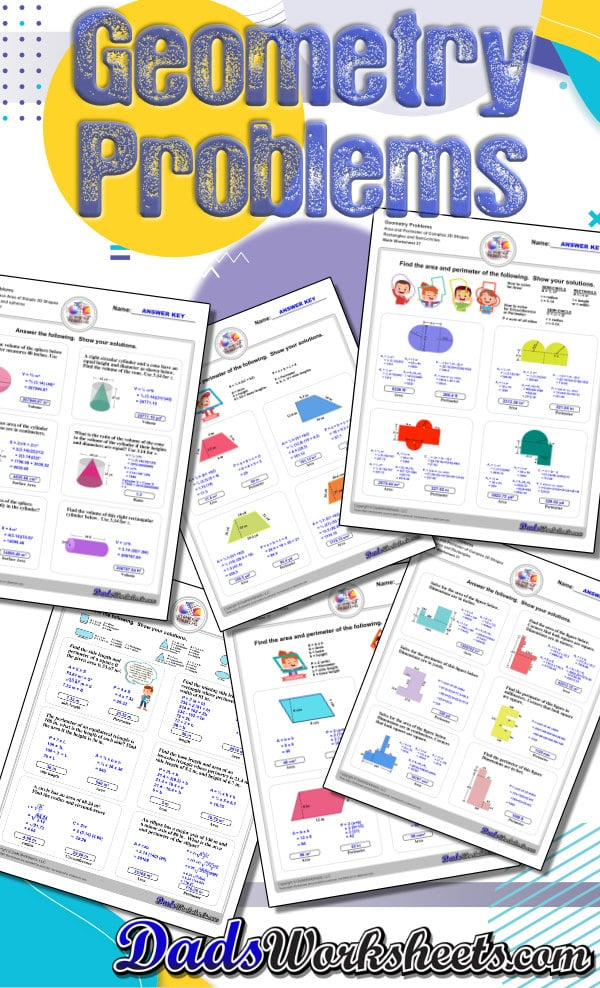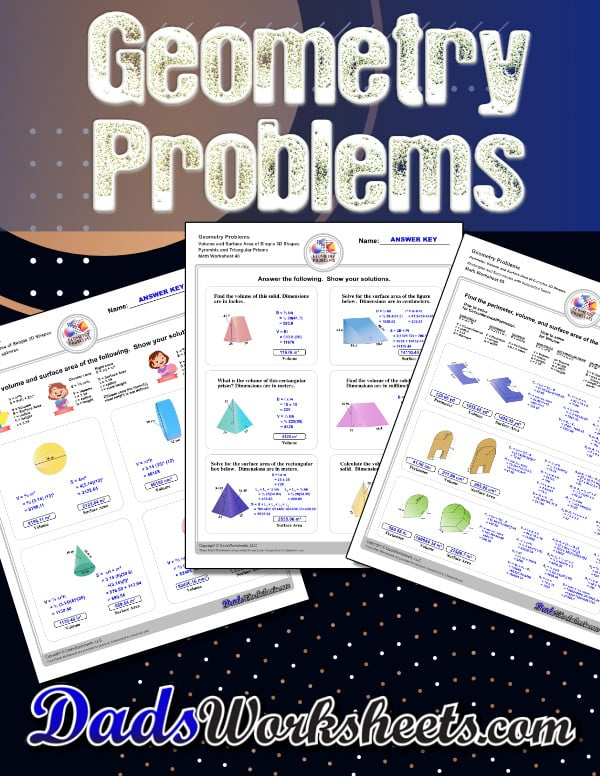# Geometry Worksheets

The geometry worksheets on this page cover topics introductory topics introduced in 5th grade as part of typical math curricula including identifying shapes, math for angles and other assignments. More advanced topics including area, perimeter and volume introduce problem solving skills appropriate for 9th and 10th grade geometry practice, or practice for visual problems on standardized tests. All worksheets include answers keys, and many topics include geometry reference charts for concept help.

## Shapes and Solids Worksheets

### 52 Shapes and Solids Worksheets

This page has dozens of printable shapes worksheets for identifying and naming 2D and 3D shapes. Activities for kindergarten and preschool age students include identifying counts of faces, edges and vertices. Students also learn to identify the shapes of real world objects, and practice worksheets include shape spelling and shape crossword puzzles.

Shapes Worksheets

## Transformation Worksheets

### 32 Transformation Worksheets

These transformation math worksheets practice rotation, reflection and translation transformations of geometric shapes on a coordinate plane. These worksheets are perfect practice exercises for 5th, 6th and 7th grade geometry students.

Transformation Worksheets

## Pythagorean Theorem

### 28 Pythagorean Theorem Worksheets

These Pythagorean Theorem worksheets require students to find the different sides of triangles using Pythagoras's theory establishing a relationship between the triangles proportions. Pythagorean Theorem Modeling V2

Pythagorean Theorem Worksheets

## Area And Perimeter

### 20 Area And Perimeter Geometry Worksheets

The area and perimeter worksheets on this page start with requiring students to calculate area and perimeter of basic shapes such as triangles, squares, circles and ellipses. Additional worksheets with compound shapes require students to calculate missing dimensions and use problem solving skills and strategies to calculate area and perimeter.

Area And Perimeter Worksheets

## Angles of Triangles

### 20 Angles in Triangles Worksheets

Angles of triangles worksheets, including finding missing angles by summing the interior angles, exterior angles of triangles and angle bisectors. Angles Of Triangles Missing Exterior Angle V1

Angles in Triangles Worksheets

## Centers of Triangles

### 24 Centers of Triangles Worksheets

Center of triangle worksheets for practice finding the centroid of a triangle, orthocenter of a triangle, and circumcenter of triangles. Also includes concept and vocabulary worksheets.

Triangle Center Worksheets

## Volume and Surface Area

### 32 Volume and Surface Area Geometry Worksheets

The volume and surface area worksheets on this page start with requiring students to calculate area and perimeter of basic solids such as cubes, prisms, cones and spheres. Additional worksheets with compound shapes require students to calculate missing dimensions and use problem solving skills and strategies to calculate volume and surface area.

Volume and Surface Area Worksheets

## Measuring Angles with Protractors

### 40 Protractor Worksheets

Measuring angles with a protractor worksheets, including blank printable protractor PDFs and detailed instructions on how to use a protractor to measure different types of angles.

Protractor Worksheets

## Complementary Angle Geometry Worksheets

### 12 Basic Geometry Worksheets

Geometry worksheets with subtraction problems for finding complementary angles (Angles whose sum is 90 degrees).

Complementary Angle Geometry Worksheets

## Supplementary Angle Geometry Worksheets

### 12 Basic Geometry Worksheets

Geometry worksheets with subtraction roblems for finding supplementary angles (Angles whose sum is 180 degrees).

Supplementary Angle Geometry Worksheets

## Parallel, Perpendicular, Intersecting

### 20 Basic Geometry Worksheets

Labelling lines as parallel, perpendicular or intersecting. Includes line shapes that imply intersection.

Parallel, Perpendicular, Intersecting

## Identifying Angles Geometry Worksheets

### 24 Basic Geometry Worksheets

Label angles as right, obtuse or acute. Optionally estimate or use a protractor to measure the actual angles.

Identifying Angles Geometry Worksheets

## Identifying Shapes Geometry Worksheets

### 24 Basic Geometry Worksheets

These geometry worksheets require students to identify and name basic quadrilaterals, triangles and polygons.

Identifying Shapes Geometry Worksheets

## Area and Perimeter of Rectangles

### 12 Basic Geometry Worksheets

The problems on these geometry worksheets require students to calculate the area and perimeter of rectangles given their dimensions. The worksheets also work great for multiplication practice!

Area and Perimeter of Rectangles

## Circles - Diameter and Radius

### 16 Basic Geometry Worksheets

Worksheets to calculate radius from diameter, diameter from radius, or radius and diameter from area or circumference.

## Circles - Area and Circumference

### 16 Basic Geometry Worksheets

Geometry worksheets with problems calculating a circle's area and circumference.

Circles - Area and Circumference

## Circles - Relationhips

### 8 Basic Geometry Worksheets

Calculate the radius, diameter, area and circumference given one circle fact.

Circles - Relationhips

## Geometry Worksheets

The basic geometry worksheets in this section cover a number of basic areas of knowledge in this deep section of mathematics. Geometry is one of the first topics where basic arithmetic is applied to more advanced mathematical concepts. The concepts of angular measurement, complementary and suplementary angles, measurement of angles within triangles, the calculator of area of shapes (including circles) are all a promising playground for applying the math skills learned at early stops on a students mathematical journey

### Angles Geometry Worksheets Topics

The geometry worksheets in this section start out with applying basic addition and subtraction skills to determine supplementary and complementary angles. Additional worksheets here also deal with idenfifying parallel or intersecting lines, and identification of right angles. While these worksheets a suitable for estimating common angle dimensions, some of these worksheets can also be used as practice for measuring angles with a protractor (the correct angle measurement is given in the answer key for each geometry worksheet).

### Shapes Geometry Worksheets Topics

One area of knowledge in geometry is simple identification of shapes, and learning the names for shapes with a certain number of sides is a rote activity. For quadrilaterals, being able to differentiate between a parallelogram (including the special case of the square), rhombus and a trapezoid are important skills. For triangles, there are geometry worksheets that help memorize the types of triangles (equilateral, right, isosceles, as well as acute versus obtuse.) .Additional worksheets in this section deal with finding the perimeter and area of rectangles.

### Circle Geometry Worksheets Topics

Circles are a unique species of geometric shape, and the geometry worksheets in this section introduce the basic equations for calculating area and circumference of a circle. Problems that explore the relationships between the diameter and the radius are also provided, giving plenty of opportunity to explore the relationships between these values and pi and the area and circumference of a circle.